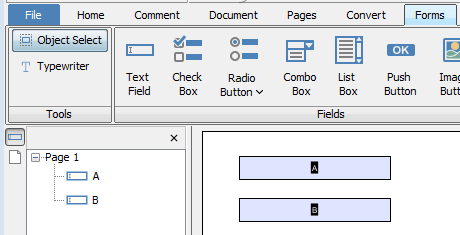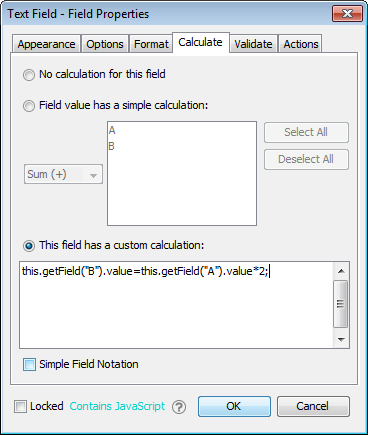Q: I want to create a custom JavaScript calculation on my form fields other than the predefined simple calculations, do you have an example for that?

A: Here is a simple example of how to create custom JavaScript calculations. In this article, we will create 2 fields “A” and “B” and compute the value of field B from the value of field A, with B = 2 * A.

Follow the steps / instructions below:

1. In PDF Studio, go to the “Forms” tab and click on Text Field

2. Create two text fields called A and B.3. Right click on field B and select Properties

5. Click Calculate and select “This field has a custom calculations”

6. Put the following script under text box:

`this.getField("B").value=this.getField("A").value*2;`7. Hit OK

8. Exit form editing (by clicking on the Home Tab)

9. Start testing the calculations.